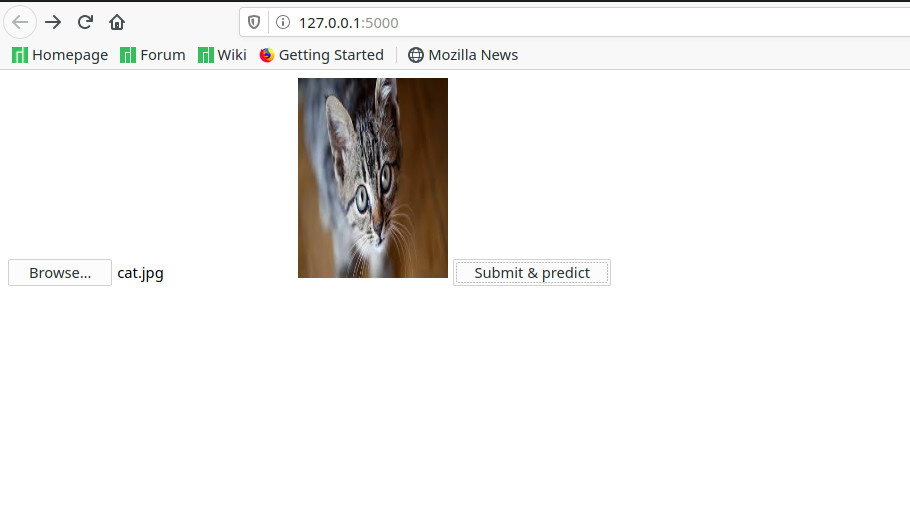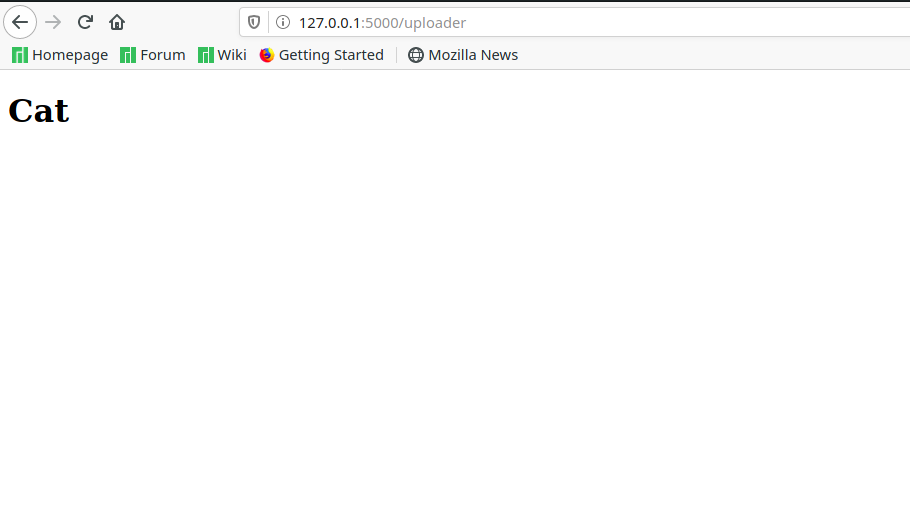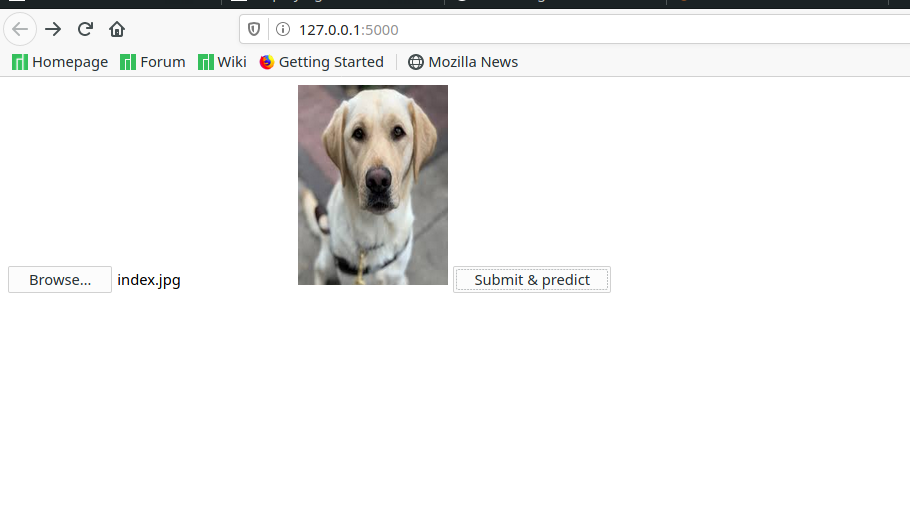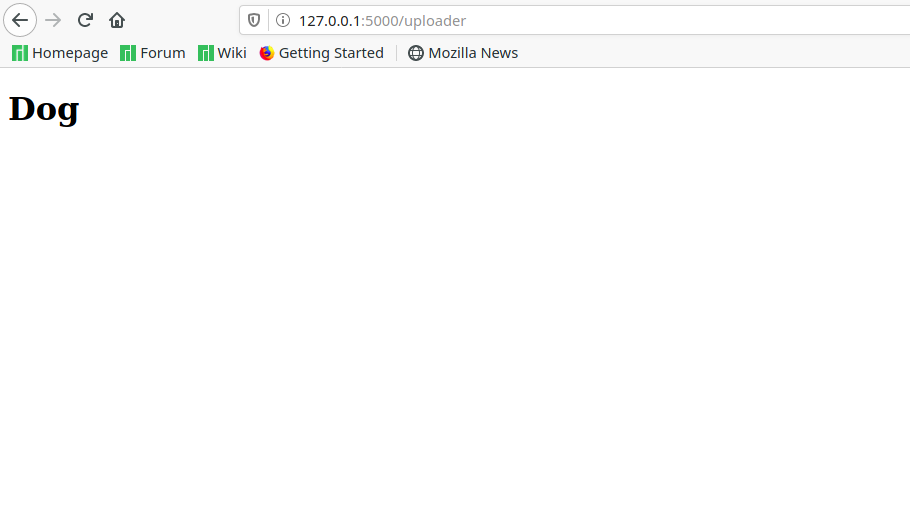# Deploying a TensorFlow 2.1 CNN model on the web with Flask

When starting to learn Machine Learning, one of the biggest issues people face is deploying whatever they make to the web for easier demonstration/use. This article will help those beginners bridge the gap between creating a TensorFlow model and deploying it on the web with Flask and hopefully gain some insight on the issues TensorFlow and Flask have.

Creating a Model
First we will have to train our model using TensorFlow and Keras’s ImageDataGenerator. For this, We have downloaded 12500 images of Cat’s and 12500 images of dogs respectively. To train a model using ImageDataGenerator first install `keras` and `tensorflow`,

`pip install keras`
`pip install tensorflow`

We have trained a model using transfer learning from `InceptionV3` model. For a tutorial on transfer learning visit this link. After the model has been trained, we will need to execute the following command which creates a model folder of the trained model. (Before TensorFlow 2.0, a model file was created instead of a model folder).

 `model.save(``'Name_of_model'``) `

Then, we will have to install Flask. Flask is a micro-framework in Python which is extensively used to deploy ML models on the web, we will have to install flask using the following command.

`pip install flask`

Coming to the webpage we want to build. Create a HTML page according to your preference, we have created a basic webpage with a button to upload an image and a button to predict what the image is. This code sends the image as a post request and stores it in the folder “uploaded/image/” where a prediction is made from here. Courtesy of Shubham S. Naik from Stack Overflow. The code is divided into 2 files, namely a .js file an a .html file. Copy the below code into a file named `upload.html` and save it in a folder named templates.

 ` ` ` `  `<``html``> ` ` `  `<``head``> ` `    ``<``link` `class``=``"jsbin"` `href``=``"http://ajax.googleapis.com/ajax/libs/jqueryui/1/themes/base/jquery-ui.css"` `        ``rel``=``"stylesheet"` `type``=``"text/css"` `/> ` `    ``<``script` `class``=``"jsbin"` `src``=``"http://ajax.googleapis.com/ajax/libs/jquery/1/jquery.min.js"``> ` `    ``<``script` `class``=``"jsbin"` `src``=``"http://ajax.googleapis.com/ajax/libs/jqueryui/1.8.0/jquery-ui.min.js"``> ` `    ``<``meta` `charset``=``utf``-8 /> ` ` `  `    ``<``script` `src``=``"{{ url_for('static', filename='upload.js') }}"``> ` ` `  `    ``<``style``> ` `        ``article, ` `        ``aside, ` `        ``figure, ` `        ``footer, ` `        ``header, ` `        ``hgroup, ` `        ``menu, ` `        ``nav, ` `        ``section { ` `            ``display: block; ` `        ``} ` `    `` ` ` ` ` `  `<``body``> ` `    ``<``form` `action``=``"http://127.0.0.1:5000/uploader"` `method``=``"POST"` `enctype``=``"multipart/form-data"``> ` `        ``<``input` `type``=``'file'` `name``=``'file'` `onchange``=``"readURL(this);"` `/> ` `        ``<``img` `id``=``"blah"` `src``=``"#"` `alt``=``"your image"` `/> ` `        ``<``button` `type``=``"submit"``>Submit & predict ` ` `  `    `` ` ` ` ` `  ` `

Copy the below code into a file named `upload.js` and save it in a folder called static.

 `function` `readURL(input) { ` `if` `(input.files && input.files) { ` `    ``var` `reader = ``new` `FileReader(); ` ` `  `    ``reader.onload = ``function` `(e) { ` `        ``\$(``'#blah'``) ` `            ``.attr(``'src'``, e.target.result) ` `            ``.width(150) ` `            ``.height(200); ` `    ``}; ` ` `  `    ``reader.readAsDataURL(input.files); ` `} ` `} `

After this is done, we will need `ImageDataGenerator` to make a prediction of an image once it has been trained. First we will load the model using

 `model ``=` `tf.keras.models.load_model(``'Name_of_model'``) `

Then, we will need to import ImageDataGenerator. Now, coming to the flask the flask code. Copy the below code into a `Code.py` file and place it in the root directory. The complete Python code is as follows:-

 `from` `flask ``import` `Flask, render_template, request ` `from` `werkzeug ``import` `secure_filename ` `from` `keras.preprocessing.image ``import` `ImageDataGenerator ` `import` `tensorflow as tf ` `import` `numpy as np ` `import` `os  ` ` `  `try``: ` `    ``import` `shutil ` `    ``shutil.rmtree(``'uploaded / image'``) ` `    ``%` `cd uploaded ``%` `mkdir image ``%` `cd .. ` `    ``print``() ` `except``: ` `    ``pass` ` `  `model ``=` `tf.keras.models.load_model(``'model'``) ` `app ``=` `Flask(__name__) ` ` `  `app.config[``'UPLOAD_FOLDER'``] ``=` `'uploaded / image'` ` `  `@app``.route(``'/'``) ` `def` `upload_f(): ` `    ``return` `render_template(``'upload.html'``) ` ` `  `def` `finds(): ` `    ``test_datagen ``=` `ImageDataGenerator(rescale ``=` `1.``/``255``) ` `    ``vals ``=` `[``'Cat'``, ``'Dog'``] ``# change this according to what you've trained your model to do ` `    ``test_dir ``=` `'uploaded'` `    ``test_generator ``=` `test_datagen.flow_from_directory( ` `            ``test_dir, ` `            ``target_size ``=``(``224``, ``224``), ` `            ``color_mode ``=``"rgb"``, ` `            ``shuffle ``=` `False``, ` `            ``class_mode ``=``'categorical'``, ` `            ``batch_size ``=` `1``) ` ` `  `    ``pred ``=` `model.predict_generator(test_generator) ` `    ``print``(pred) ` `    ``return` `str``(vals[np.argmax(pred)]) ` ` `  `@app``.route(``'/uploader'``, methods ``=` `[``'GET'``, ``'POST'``]) ` `def` `upload_file(): ` `    ``if` `request.method ``=``=` `'POST'``: ` `        ``f ``=` `request.files[``'file'``] ` `        ``f.save(os.path.join(app.config[``'UPLOAD_FOLDER'``], secure_filename(f.filename))) ` `        ``val ``=` `finds() ` `        ``return` `render_template(``'pred.html'``, ss ``=` `val) ` ` `  `if` `__name__ ``=``=` `'__main__'``: ` `    ``app.run() `

Finally we need to render a html page for the final prediction. Copy the below code and place save it as `pred.html` in templates folder.

 ` ` ` `  `<``html``> ` `<``body``> ` `<``h1``>{{ ss }} ` ` ` ` `

The final folder structure is described as follows :-
The black colored flags are folders and blue flags are files.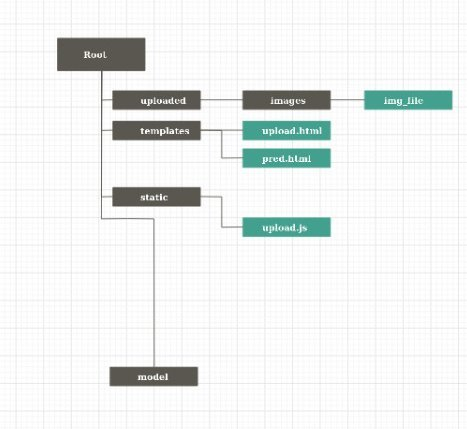Output
Finally, all we need to do is run the file code.py and go to http://127.0.0.1:5000/ to see the output